Choose Language Hide Translation Bar
Highlighted

## How to look at variability in this data

We did measurements in duplicate 96-well plates (so 96 different samples equally split into two plate). We want to estimate variability between the plates.  It seems to me that there are two important sources of variability, one related to time since one plate was measured at a different time and so all samples probably degraded equally in that plate and another related to other differences between the duplicates.

We just took the % CV for each duplicate and looked at average or median %CV. Is there a better way to look at how the plates are different with JMP? Also, It doesn't seem to me that "1-r2" (uneplained variation) of the correlation between the plates is the right way to calculate this.

1 ACCEPTED SOLUTION

Accepted Solutions
Highlighted

## Re: How to look at variability in this data

The estimate for 96 Plate = Var1B_1 is -0.063997 (below the grand average) so the total effect between the second and first plate is +0.127994. Is this result consistent with your hypothesis of degradation over time?

Learn it once, use it forever!
6 REPLIES 6
Highlighted

## Re: How to look at variability in this data

I tried to re-create your design. Instead of sample, I used two categorical factors: one for the plate columns (8 levels) and another for the plate rows (12 levels) to yield 96 samples. I added a factor for plate (2 levels).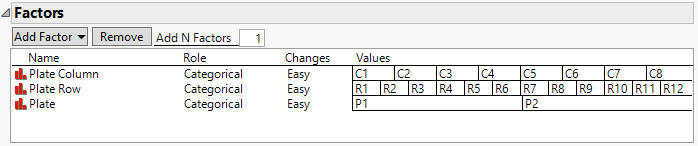I added a term for a potentila interaction effect between plate column and plate row.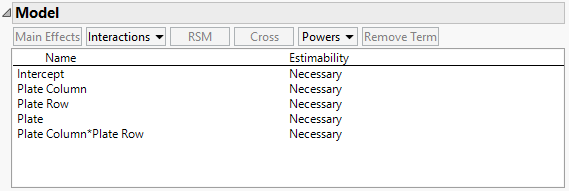I made a design for 192 runs. I made a table and sorted the runs by plate, plate row, and plate column. (I would normally randomize the runs but it is different with a plate.) I simulated your response with a simple standard normal distribution (all effects are null)to illustrate the analysis. I clicked the green triangle next to the Model table script. I clicked Run.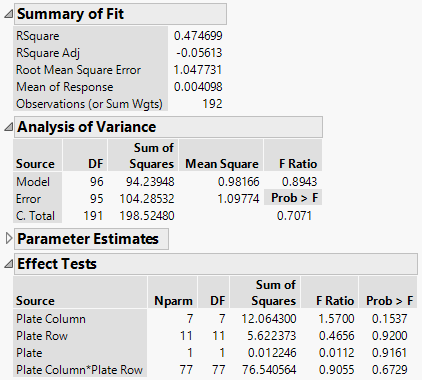Use the Effect Tests to decide if the fixed effects of the wells is statistically significant. Use the Analysis of Variance to decide if the model, as a whole, is statistically significant. Use the Root Mean Square Error (RMSE) as the pooled estimate of the sample standard deviation.

Learn it once, use it forever!
Highlighted

## Re: How to look at variability in this data

Thanks Mark.

Note: the duplicates are in the same location (well number) in each plate. Just in case this makes a difference for the analysis.

I tried to replicate what you did with the fit model and got the below: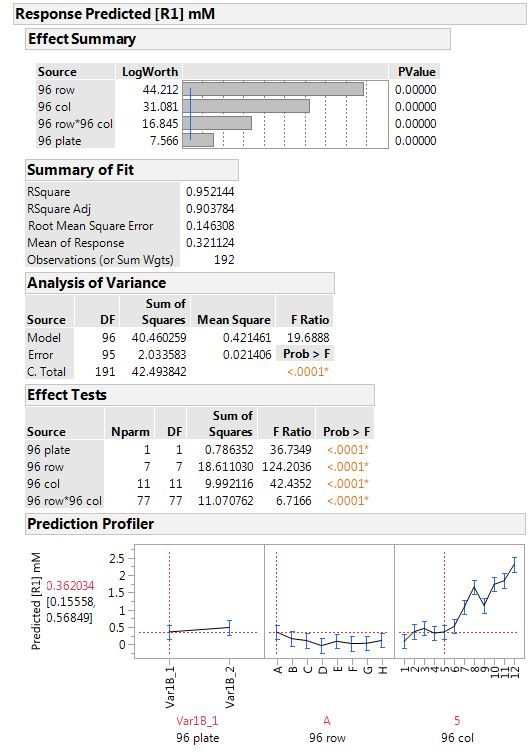I guess this says there's a significant difference between the duplicates in the two plates? are we able to say how much in comparison to well-to-well variation in each plate?  Thanks

Highlighted

## Re: How to look at variability in this data

You didn't show the Parameter Estimates outline. The estimate for 96 Plate will be the difference for the first plate from the intercept (grand average). The other plate has the negative of this estimate so the total effect (difference between plates) is twice the estimate shown.

The RMSE=0.146308 is the standard deviation of the replicates.

Learn it once, use it forever!
Highlighted

## Re: How to look at variability in this data

oops here it is: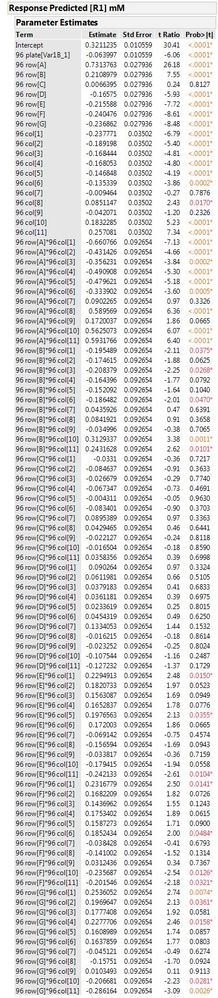Highlighted

## Re: How to look at variability in this data

The estimate for 96 Plate = Var1B_1 is -0.063997 (below the grand average) so the total effect between the second and first plate is +0.127994. Is this result consistent with your hypothesis of degradation over time?

Learn it once, use it forever!
Highlighted

## Re: How to look at variability in this data

Thank you Mark. This helps a lot.

Article Labels

There are no labels assigned to this post.